GET THE APP

Robust Detection of Outlier Samples and Genes in Expression Datas# Journal of Proteomics & BioinformaticsOpen Access

Research Article - (2016) Volume 9, Issue 2

# Robust Detection of Outlier Samples and Genes in Expression Datasets

Ahmad Barghash1,2, Taner Arslan1 and Volkhard Helms1*
1Center for Bioinformatics, Saarland University, Saarbruecken, Germany
2Saarbruecken Graduate School of Computer Science, Saarbruecken, Germany
*Corresponding Author: Volkhard Helms, Center for Bioinformatics, Campus E2 1, R. 315, P.O. Box 15 11 50, D-66041 Saarbrucken, Germany, Tel: +49 681 302 70701, Fax: +49 681 302 70702

## Abstract

Expression and methylation datasets are standard genomic techniques and an increasing number of computational methods are implemented to aid in analyzing the huge and complex amount of generated data. Such generated datasets often contain a sizeable fraction of outliers that cause misleading results in downstream analysis. Here, we present a comprehensive approach to detect sample and gene outliers in expression or methylation datasets. The core algorithms detected most outliers that were artificially introduced by us. Sample outliers detected by hierarchical clustering are validated by the Silhouette coefficient. At the gene level, the GESD, Boxplot, and MAD algorithms detected with f-measure of at least 83% the simulated outlier genes in non-intersected distributions. This combined approach detected many outliers in publicly available datasets from the TCGA and GEO portals. Frequently, some functionally similar genes marked as outliers turned out to have outlier observations in common samples. As such cases may be of special interest, they are labeled for further investigations. Expression and DNA methylation datasets should clearly be checked for outlier points before proceeding with any further analysis. We suggest that already 2 outlier observations are enough to label an outlier gene as they are enough to ruin a perfect co-expression. Besides, outliers might also carry useful information and thus functionally similar outliers should be labeled for further investigation. The presented software is freely available via github

Keywords: Outlier detection, Functional Similarity, GESD, MAD, Boxplot, Hierarchical clustering

#### Abbreviations

AHC-ED: Average Hierarchical Clustering based on Euclidian Distances; GSED: Generalized Extreme Studentized Deviate; MAD: Median Absolute Deviations about the Median

#### Introduction

Monitoring gene expression can aid in cancer classification  and in identifying clinically-relevant tumor subgroups . Additionally, profiling of gene expression is one key approach for finding new biomarkers and therapeutic targets for different cancer types . Several data portals such as the Gene Expression Omnibus (GEO)  and The Cancer Genome Atlas (TCGA) now provide convenient access to thousands of normalized expression datasets for most cancer types. However, automatic processing of these data is complicated due to the occasional appearance of outlier samples or outlier genes in such large datasets.

In simple words, an outlier is an observation that deviates “too much” from other observations. Detecting outliers might be important either because the outlier observations are of interest themselves or because they might contaminate the downstream statistical analysis. In the field of gene expression, an outlier can be an abnormal sample that deviates significantly from the other samples in its class. One common reason for this is mislabeling, where accidently a sample of one class might be falsely assigned to another one. Mislabeled samples might then reduce the distinction between true dataset classes. On the other hand, an outlier might also be a gene with abnormal expression values in one or more samples from the same class. In the case of cancer, this may reflect that this patient or his/her disease is a special case. Hence, it is important to identify outliers in expression datasets and, depending on the type of analysis to be performed, to consider whether this data should be removed .

Recently, several methods have been proposed for outlier detection in microarray data that used, for example, principle component analysis and estimation of Mahlanobis distances , a hybrid evolutionary algorithm , cross validation of an SVM classifier , a Gene Tissue Index , or the OASIS methods . Some studies predicted outliers for the sake of filtering while others predicted them for further analysis. To the best of our knowledge, no approach so far detects sample as well as gene outliers with a set of suitable filters to validate the detection.

In this work, we propose and test a simple approach that combines multiple established methods to detect outlier samples or genes in expression and methylation datasets. Average hierarchical clustering is used to detect outlier samples and the clustering is later validated using the Silhouette coefficient. To detect outlier genes we use the three algorithms GESD , Boxplot, and MAD . As there is no fixed threshold for outlier observations required to label a gene as an outlier, and it is dataset size-dependent, we introduce in this work the usage of co-expression feature to address this issue.

We note that, some outlier genes might carry useful information behind the outlier observations. For this, we introduce functional similarity of abnormal genes as an additional filter for outlier genes. Semantic similarity is analyzed using tool GOSemSim . If genes show outlier expression and share high functional similarity with other detected outliers, they are kept for further analysis.

#### Materials and Methods

In this work we introduce a hybrid technique based on established algorithms to detect outlier samples and genes in expression datasets. Samples are denoted as outliers if they deviate more than a certain threshold in Euclidean distance from other samples in the same class (tumor/normal). The threshold is not fixed but dataset-dependent. To find outlier samples, we used average hierarchical clustering based on Euclidean distance (AHC-ED). Subsequently, we use the Silhouette measurement to validate the quality of the clustering. On the other hand, genes are labeled as outliers if their reported expression values contain outlier observations that pass a suggested threshold and if they share no significant functional similarity with other detected outlier genes. If the expression of one gene follows a normal distribution, we use the Generalized Extreme Studentized Deviate algorithm (GESD) . If the gene expression data does not follow a normal distribution, then we apply the two distribution-free algorithms Boxplot and Median Absolute Deviations about the median (MAD) . We additionally test functional similarity within outlier genes using GOSemSim . If such gene pairs are found, we check whether their outlier observations are detected in common samples. Functionally dissimilar outlier genes are later marked for removal. The pipeline is illustrated in Figure 1.

Datasets

To test the hybrid approach just introduced, we generated four simulated expression datasets with known outliers at the gene and sample levels. Additionally, we tested the workflow on a public colon cancer dataset with known outliers published by . Subsequently, we applied our approach to predict outliers in public datasets of colon cancer, glioblastoma multiforme (GBM), ovarian cancer (OV), and liver cancer obtained from The Cancer Genome Atlas (TCGA) and the Gene omnibus (GEO) databases.

Data with known outliers

Initially, we generated four simulated datasets with known outlier samples or genes in a scenario that resembles a typical cancer dataset. Each dataset contains two clearly distinguishable classes of samples. Thus outlier samples either do not match the majority of samples in either of the two classes or are simply mislabeled. On a different manner, a gene is considered an outlier if it presents a clear uneven simulated behavior within either class. In the literature, the overall shape of the distribution of gene expression levels is typically not explicitly mentioned. Several studies apply tests for normality to check whether the data follows a Gaussian distribution [14-16]. We speculated that in rare cases, the distribution of gene expression might also follow a Poisson distribution. Thus, we created two simulated datasets that obey either a Gaussian distribution or a Poisson distribution.

The simulated datasets contained 100 samples distributed equally to two classes and 1000 genes each. The first 50 samples belonged to class 1 (C1) and the other 50 to class 2 (C2). The form of the first two datasets (SDS1/2) is the same and they were both used for identification of sample outliers. At first, the first 900 rows are drawn from the same distribution for both classes but the remaining 100 were drawn from different distributions. In SDS1, 900 rows were drawn from the normal distribution N(0,22) (see equation 1 by setting μ=0 and σ=2) but the remaining 100 were drawn either from N(10,12) or N(20,12) for samples of classes C1 and C2, respectively. In SDS2, the first 900 rows were drawn from the same distribution like in SDS1 but the remaining 100 were drawn from distributions N(10,22) and N(15,12) for samples of classes C1 and C2, respectively. SDS2 represents clearly overlapping classes. Later, samples 10, 15, and 20 from class 1 were switched with samples 60, 65, and 70 from class 2 as a set of mislabeled samples in both datasets. Additionally, the last sample from each class was replaced by one drawn either from N(25,12) or N(30,12) in classes 1 and 2, respectively, to create clear outlier samples (Figure 2).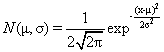(1)

The third and fourth datasets (SDS3, SDS4) were used for identification of outlier genes. Each had 50 known outlier genes with outlier values at the same positions in classes C1 and C2. In SDS3, the 950 non-outlier genes were filled from Gaussian distributions N(0,22) and N(15,32) for classes C1 and C2, respectively. Regarding the outlier genes, 45 points followed the class rules and the other five were drawn from N(12,12) and N(2,12) for classes 1 and 2, respectively. To overcome the randomness in the created distributions, we generated 100 arrays in the form of SDS3 and passed them later to the outlier detection algorithms. All normal distributions for non-outlier points were controlled by Shapiro tests with p-value threshold of 0.1.

The 950 non-outlier genes in SDS4 were filled from a Poisson distribution with λ equal to 2 or 3 for classes 1 and 2, respectively. To simulate outliers in the remaining 50 genes, we filled 45 out of 50 points in each gene with values from the class distribution like before but the remaining five points were filled from Poisson distributions with λ equal to 3 or 0.5 for classes 1 and 2, respectively. Here, we used minimum chi-square estimation  to fit the generated distributions and accepted those with an upper p-value threshold of 0.0001.

As a further test on an experimental dataset, we considered an extensively studied experimental dataset with documented outlier samples in colon cancer . This dataset has 22 normal and 40 tumor samples. Several classification algorithms were previously applied to this dataset and suggested many outliers and misclassified samples between tumor and normal [5,7]. For example, Alon et al.  used a two-way clustering algorithm and found eight misclassified samples. Furey et al.  and Moler et al.  used linear SVM classifiers and found six misclassified samples. Also, Li et al.  found 6 misclassified samples using a genetic algorithm. Albert Shieh et al.  found the nine outlier samples using PCA. Overall, nine samples can be considered as confirmed outliers (T2, T30, T33, T36, T37, N8, N12, N34, N36) and were used here to test our outlier detection approach.

Application to public datasets

After validating the workflow shown in Figure 1 on the test datasets with known outliers, we applied this hybrid technique to detect unknown outliers in public cancer datasets downloaded from TCGA for colon, GBM, and OV cancers and from GEO for liver cancer (Table 1). In GEO, a sample description is included in the main dataset page.This is not the case with TCGA. In TCGA datasets, normal and tumor samples can be distinguished by their barcodes. The barcode has several parts separated by hyphens. The third part - with two digits number and a character - describes the sample. Numbers from 0-9 label cancer samples while numbers from 11-19 label normal samples.

Dataset Raw data type Normal samples Tumor samples Download data # Genes # Genes obeying normal distribution
COAD Expression Agilent 7 143 08.Feb.2013 11687 5971
GBM expression Agilent 10 594 04.Apr.2013 17430 2820
OV expression Agilent 7 591 07.Apr.2013 17436 4112
Liver expression (GSE14520) Affymetrix 239 247 01.July.2013 12701 N:1144
T:1791
COAD Methylation Illumina Infinium HumanMethylation27 0 129 28.Apr.2013 11633 1082
GBM Methylation Illumina Infinium HumanMethylation27 0 294 28.Apr.2013 10256 98
OV Methylation Illumina Infinium HumanMethylation27 8 597 28.Apr.2013 7876 14

Table 1: Datasets.

Detection algorithms

To detect outlier samples, we cluster samples using the average hierarchical clustering based on Euclidean distance. Subsequently, we use the Silhouette measurement as a measure of the quality of clustering. Based on the clustering vector and the set of distances, the algorithm calculates the average dissimilarity of a point to its current class a(i) and the lowest dissimilarity of the point to other classes b(i). The combination of dissimilarity according to equation 2 measures how well elements fit into their clusters. S(i) ranges between (-1,1) where 1 indicates a better fit to the current cluster and -1 means that the point actually belongs to the other class or a so called neighboring cluster.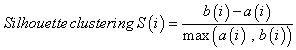(2)

The Silhouette coefficient for the objects of one cluster is defined as the arithmetic mean of the Silhouette values of all objects.

To detect outliers at the gene level, we use the 3 algorithms GESD, Boxplot, and MAD. GESD was developed to detect one or more outliers in a dataset assuming that the body of its data points comes from a normal distribution . Precisely, this algorithm calculates the deviation from the mean for every point,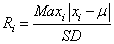(3)

and then removes the point with the maximum deviation at each iteration. This process is repeated until all outliers that fulfill the condition Rii are identified where λ is the critical value calculated for all points using the percentage points of the t distribution.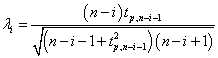(4)

GESD and its predecessor ESD will always mark at least one data point as outlier  even when there are in fact no outliers present. Therefore, using GESD to detect outliers in microarray data must be accompanied with a threshold of outlier allowance where a certain amount of outliers are detected before marking a gene as an outlier. The GESD method is said to perform best for datasets with more than 25 points . Additionally, the algorithm requires the suspected amount of outliers as an input. The default in this work is half of the tested size.

Besides GESD, we additionally use the well-known Boxplot method. This is also a non-parametric algorithm but can detect outliers without pre-assumption about the underlying statistical distribution. Boxplot calculates five key points for plotting; two extremes (whiskers), upper and lower hinges (quartiles), and the median. Data points outside the hinges are labeled as possible outliers. As the quartiles and whiskers are not distribution-driven (related), Boxplot normally suggests many points as outliers and thus datasets might extremely shrink . Therefore, we use this algorithm for gene expression data sets that failed the normality test and we suggest an allowed margin of outliers.

The last algorithm we apply is the MAD algorithm. This algorithm does not rely on the variance or standard deviation and thus it assumes no special statistical distribution of the data similar to Boxplot. Here, first the raw median for each gene is calculated over all samples. Then the median absolute deviation (MAD) of data points from the raw median is calculated as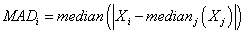(5)

Data points with maximum MAD are labeled as possible outliers.

Hereafter, in this manuscript, we will label as outliers those genes with at least two outlier values (see below). We will use the GESD algorithm only if the gene expression follows a normal distribution and expression data is available from at least 25 samples. For other genes we use MAD and Boxplot to detect outliers and we accept decisions if they match for at least 2 of the outlier observations.

The analysis in this work was completed in R-cran mainly using the parody package. To make it publicly available, the same workflow was implemented as a GUI Python tool for outlier detection. This tool offers special implementations of the algorithms mentioned in this work and some other features. AHC-ED followed by Silhouette are used to identify outliers at the sample level while GESD, Modified z-score , adjusted Boxplot  and the median rule  are used at the gene level. Once the outliers are detected, the tool offers to group outliers on the basis of their co-expression, functional similarity, or their KEGG pathway participation. The user is asked almost at every step to input his confidence thresholds. The tool provides dataset statistics, detection statistics, and outlier similarity statistics while allowing the user to export the findings at the different stages. Related Figures are generated and saved to the disk automatically where needed. The tool is available at GitHub via the link: https://github.com/TanerArslan/outlier-detection

#### Results

As a start we illustrate the effect of two outlier data points on co-expression analysis.

Effect of two introduced outlier observations

Co-expression analysis is important for suggesting functional gene-gene interactions. Thus, one may wonder how many outliers are needed to ruin a known co-expression. To test this, we randomly picked one gene each from the 4 public cancer expression datasets studied in this work and introduced two outliers to it. Then we compared the correlation of expression between its raw expression and its modified one. The magnitude of their deviation from the mean was measured in multiples of the standard deviation (SD). Perturbations ranged from 2SD to 12SD. Figure 3 illustrates the effect on genes with different numbers of samples

Figure 3 illustrates that introducing only 2 outlier data points with 2 standard deviations from the mean in samples with 143 to 594 data points decreases the auto-correlation of the data from 1 to 0.76-0.94 depending on the size of the dataset. Hence, already few undetected outliers may have a large effect on the biological interpretation of the data. Based on this result, and knowing that some outlier detection algorithms have a marginal error of one outlier, we consider in the following genes as outlier genes if they have at least 2 outlier values.

Detecting outliers in data with known outliers

Next, we tested the outlier detection approach illustrated in Figure 1 using four datasets of simulated expression. SDS1/2 were generated to have two classes to simulate cancer and normal classes. Each class contained a pure outlier sample and three mislabeled samples. In SDS3/4, 50 outliers were distributed among the members of the two classes with five outlier points out of 50 in each class (Figure 4).

Detecting known outlier samples in simulated datasets

Here, we first tested the sensitivity of the clustering algorithms using simulated expression data. The first 900 rows in the two classes were filled from the same distribution and the remaining 100 rows were filled from different distributions for the two classes. The outlier sample detection module successfully classified samples into the two main classes even when only 10% of these rows are different between classes C1 and C2. Additionally, the module detected the two pure outlier samples and labeled them as a third class away from the other two. Finally, the module successfully managed to detect the mislabeled samples 10, 15, 20 from the first class and 60, 65, 70 from the second class and mapped them to the correct classes.

Then, we tested the quality of clustering using the Silhouette method. We found that the two clusters are well separated with an average distance of 0.36 within the SDS1 clusters and 0.14 in SDS2 with semi-nested classes, see Figure 5.

Since this first test was very satisfactory, we then tested the stability boundaries of this detection method. First, we varied the proportion of the SDS1 dataset that is being filled from the same distributions. Here we performed 3 runs filling 950, 975, or 990 rows from the raw distribution and filling the remaining rows from the class specific distributions as before. Then we clustered the samples using AHC-ED and tested the clustering using Silhouette coefficients. In all runs, AHC- ED successfully clustered the samples pointing to the outliers and to the mislabeled ones. Silhouette confirmed the clustering result but with a continuously decreasing average width S(i) of 0.23, 0.14, and 0.07 on average.

As a final test, we filled the differing parts from distributions that have a larger overlap: N(0,12) as raw distribution and N(8,12) and N(9,12) for classes C1 and C2, respectively. Again we tested the four class proportions (900/100, 950/50, 975/25, 990/10) as in the first analysis. Now, Silhouette did not validate the clustering up from the second run, returning negative S(i) width, because of the frequent mislabeled samples and because of treating small differences as noise. Generally, the average Silhouette width was lower than in the first test.

Detecting known outlier genes in simulated datasets

For testing the outlier gene detection module, we used the three algorithms GESD, MAD, and Boxplot to identify simulated outliers in 100 generated datasets in the form of SDS3. Each outlier gene was modeled to have 5 known outlier values out of 50 points. We observed that the GESD algorithm was able to detect at least four out of five outlier values in 46 out of 50 outlier genes on average. In contrast, MAD and Boxplot on average detected four out of five outlier points in only 33 and 34 genes, respectively, and some outlier points of the other outlier genes. On average, 31 outlier genes were commonly detected by all algorithms as listed in Table 2. For comparison, we also tested the Rousseeuw method  to detect outliers in our simulated datasets but its performance was much lower less with 14/50 outliers on average.

GESD 46
Boxplot 33 34

Average of commonly detected outliers by GESD, Boxplot, and MAD algorithms in 100 simulated datasets of the SDS3 form. An outlier is considered as correctly detected if four out of five outlier values are detected from the other 50. DS3/4 has in total 50 outlier genes out of 1000.

Table 2: Detection results of simulated gene outliers.

To test the stability of the detection module, we then performed 3 runs filling the dataset with more intersected distributions each time. In each run we created 100 datasets with 50 outliers each and calculated the average detection of the different algorithms. We found that the GESD detection was more stable than Boxplot and MAD but still failed in the last case showing strong overlap. Table 3 lists the distributions used in each run and the detection results.

Approximate Intersection Class' Distributions Outlier distribution Detection Result
1SD C1: N(0,22)
C2: N(5,12)
C1: N(10,22)
C2: N(11,12)
GESD: 45±
Boxplot: 37±
2SD C1: N(0,22)
C2: N(5,12)
C1: N(8,22)
C2: N(10,12)
GESD: 30±
Boxplot: 18±
3SD C1: N(0,22)
C2: N(5,12)
C1: N(6,22)
C2: N(9,12)
GESD: 10±
Boxplot: 4±

Lists of all distributions used in different runs creating matrices of simulated expression.

Table 3: Distributions of simulation datasets.

In the datasets following a normal distribution, all three algorithms detected the outliers with good accuracy unless the distributions overlapped to a major extent. To describe the accuracy, we calculated precision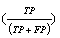, recall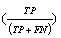and f-measure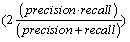accuracy measures. As explained in , accuracy measures in prediction and classification approaches emphasize the role of unexpected predictions (precision) or the role of missing predictions (recall). Along the same lines, F-measure is frequently calculated to merge the precision and recall decisions. In this sense, we consider the known outliers correctly predicted by the algorithms as “True positives (TP)” and the missed known outliers as “False negatives (FN)”. Hence, recall for the first runs of the disjoint distributions was calculated as 90%, 74%, and 72% for the GESD, Boxplot, and MAD results, respectively. On the other hand, the algorithms detected at most one additional outlier observation in non-outlier genes (which we did not introduce). Such cases could be considered “False positives (FP)”. However, no gene contains two such outlier observations which suggest perfect precision. The F-measure calculated for GESD, Boxplot, and MAD was 94%, 85%, and 83%, respectively.

However, the algorithm detected only few outliers in SDS4 following a Poisson distribution what is rarely the case in gene expression datasets. In that case, GESD detected on average 46% of the outlier points in 16 out of 50 genes and failed to detect any outlier point in the rest. MAD detected 46% of the outlier points in only 3 out of the 50 outlier genes. Boxplot detected only 23% of the outlier points in only 6 out of the 50 outlier genes. This indicates that the algorithms are most robust to detect outliers in expression datasets following more or less a normal distribution.

We now summarize the main decisions taken when establishing the workflow of Figure 1 that is implemented in the provided software package. Even in apparently “well behaved” distributed normal distributions, all algorithms detected some less significant outliers (on average one for each gene). More of such insignificant outlier values can be found in real datasets (data not shown). Therefore, we suggest that only genes with at least two outlier observations should be labeled as outliers. We experienced in our analysis that GESD is powerful in detecting outliers in data sets following Gaussian distribution. We also found that Boxplot is a quite restrictive algorithm and places many points outside of the whiskers. Therefore we suggest to implement the GESD decision in data following a normal distribution (Shapiro test p-value >0.1) and to accept the decision of Boxplot and MAD for other genes only if they match the positions of at least two outlier observations.

Detect outlier samples in public datasets with known outliers

Next, we tested the outlier sample detection module using a public dataset for colon cancer with known outlier samples in normal and tumor classes . Normal and tumor classes were treated separately. Average hierarchical clustering found 8 out of the 9 reported outlier samples and placed them on the far left in the dendrograms, see Supplemental Figure 1.

Detect outliers in public data sources

Then, we applied the established workflow to detect outliers in datasets from the public sources TCGA and GEO. At the gene level we checked the normality using Shapiro test as a precondition.

Genes with outlier behavior might actually carry useful information behind the outlier values. Therefore, as a last filter, we tested whether the genes with outlier behavior belong to a functional group by analyzing Gene Ontology (GO) annotations using the package GOSemSim . We postulate that if two or more outlier genes show a certain degree of functional similarity and have outlier points in the same samples, then the causative outlier behavior of this functional group might be interesting to analyze and thus genes should not be discarded right away. Hence, we first needed to establish a cut-off threshold for meaningful semantic similarity. To this aim, we computed the semantic similarity between all pairs of the around 11000 human genes, see Figure 6. Based on the data shown, we suggest that 0.85 is a reasonable cut-off threshold for meaningful functional similarity

Detection of outliers in TCGA datasets

In the colon dataset, AHC-ED clustered the normal samples into one cluster distanced away from most tumor sub-clusters without detecting any clear outlier or mislabeled samples, see Figure 7. The Silhouette coefficient validated this clustering with an overall average width of 0.22 (Supplemental Figure 2). As TCGA datasets so far contain only few normal samples for most cancer types, we analyzed only the tumor samples for outlier genes. The gene expression of TCGA datasets frequently followed a normal distribution. Among these genes, GESD detected only four outlier genes with at least 2 outlier values (EIF3G, GLUD1, GSG1L, STARD6). The results of MAD and Boxplot on these genes mostly supported the GESD findings. Among the non-Gaussian genes, Boxplot detected 1692 and MAD detected 1840 outliers. 1586 genes had common outlier observations in at least two samples reported by Boxplot and MAD. Interestingly, 1163 of these outlier genes were also detected by GESD applied to the non-Gaussian expression. When searching for functionally similar outliers using GOSemSim, we found that 400 outlier genes show high pairwise functional similarity to other outliers among these 400 genes.

In the GBM dataset, AHC-ED grouped the normal samples as one of the outer clusters like for the colon dataset. Additionally, several tumor samples were clustered away from the core clusters and thus they can be labeled as outliers (Supplemental Figure 3). Overall clustering was validated using Silhouette with overall an average width of 0.22 (Supplemental Figure 4). Here we suggest that further downstream analysis will be slightly improved after removing these outlier samples. At the gene level, the expression values of 2820 out of 17430 genes followed a Gaussian distribution according to Shapiro test and GESD detected 6 outlier genes among these (C6orf151, DOCK2, EIF2S2, NPR2, PLEKHA8, SH3GL1). Among the genes with non-Gaussian body, Boxplot and MAD detected 6788 and 7130 outlier genes, respectively. Both algorithms detected that 6671 outliers had at least two outlier points in common samples. Additionally, the detection of 5032 of these genes was supported by GESD. 2325 of the 6671 outlier genes shared high functional similarities and outlier observations in at least two common samples.

In the OV dataset, normal samples were clustered together but not on the outer sides. For tumor samples, clustering resulted in many small clusters which indicates weak relations between the samples (Supplemental Figure 5). Silhouette validated this clustering with average widths of 0.47 and 0.05 in normal and tumor samples, respectively (Supplemental Figure 6). The removal of the outermost 10 samples improved the clustering only slightly. At the gene level, the expression of 4112 out of 17436 genes follows a Gaussian distribution. GESD found 8 outlier genes among the genes with Gaussian expression profiles. Boxplot and MAD found 5757 and 6067 outlier genes, respectively, of which 5659 have outlier observations in common samples. GESD supported the detection of 786 of the outlier genes. 1665 outliers shared high functional similarity and outlier observations in common samples.

Detect outliers in GEO datasets

NCBI GEO provides more cancer related datasets than TCGA. Also, GEO datasets normally contain a balanced amount of normal samples. Here we applied our hybrid approach to a liver cancer dataset with 486 samples; 239 normal and 247 tumor. Normally, samples were mostly clustered into one core cluster. However, clustering tumor samples presented at least two clear tumor clusters as shown in Figure 8. Silhouette validated these findings with an average width of 0.4 for normal and 0.03 for tumor samples (Supplemental Figure 7). Here, we suggest removing only the outliers among normal samples clustered outside the core cluster. Also, for this case, we suggest that performing further analysis to tumor clusters separately might achieve clearer results.

In this dataset where only 14% of the genes had a Gaussian expression body, we found many outlier genes in this dataset as listed in Table 4. Boxplot and MAD matched at least 2 outlier positions in 7742 and 6128 outlier genes in normal and tumor samples, respectively. We found 4541 outliers in common between normal and tumor samples. However, 4716 and 3208 outlier genes shared high functional similarity in normal and tumor samples and they had outlier observations commonly in at least 2 samples.

Tumor Normal Common
GESD 7 2 0
Boxplot 6215 7846 4636

Statistics of outlier detection in GEO HCC dataset. GSE 14520. Common refers to outlier genes detected by a specific algorithm in tumor and normal samples

Table 4: Outliers detected separately in normal and tumor samples of the HCC dataset.

Detecting outliers in DNA methylation datasets

Finally, we tested the outlier detection approach to identify outliers in 3 methylation datasets downloaded from TCGA for colon, GBM, and OV cancers. Only the OV dataset had normal and tumor samples. Out of these, only the normal samples were clustered together as validated by Silhouette (Supplemental Figure 8). At the gene level, fewer genes had a Gaussian methylation body compared to expression datasets. However, most outliers found shared high functional similarity with other detected outlier genes and thus were not removed except the case of outliers detected by MAD in the COAD dataset .

Interestingly, we noticed that the 3 algorithms matched at least two outlier positions in most of the detected outliers although only few had a Gaussian body. Additionally, at least 50% of the commonly detected outliers shared high functional similarity. The fraction of outliers detected and returned by the three algorithms is shown in Figure 9.

#### Discussion

Here we presented a new robust strategy for detecting outlier samples and genes in gene expression and DNA methylation. As outliers might carry useful information we set filters to remove only the extreme outliers while labeling interesting outliers for further analysis. We presented two modules for outlier detection working at the sample and gene levels. The outlier sample detection module consists of AHC-ED to define outlier samples and the Silhouette coefficient to validate the clustering. In the outlier gene detection module we observed that the underlying distributions of the expression or methylation play a key role in the detection process. The underlying distributions are frequently Gaussian und thus the GESD algorithm would fit for detecting outliers. This module includes two other methods (Boxplot, MAD) that detect outliers regardless of the underlying distribution found.

To validate this approach, we created several expression simulated datasets with introduced sample and gene outliers and searched them using the proposed methods. Simulation datasets were filled either from disjoint or intersected distributions. AHC-ED clustered successfully samples into two classes even in the case where less than 10% of the class rows were generated from two disjoint distributions while the rest came from the same distribution. On the other hand, the more intersected the classes are the less they can be distinguished on the basis of clustering dendrograms. AHC-ED successfully clustered samples filled from intersected distributions but with a less strong Silhouette validation compared to the completely disjoint ones. In simulated datasets, we also introduced 3 mislabeled samples and the clustering mapped them to their original classes. Two additionally introduced pure outlier samples were successfully clustered far most from other classes. Later we tested the outlier sample detection module using one colon cancer public dataset that has a set of known outlier samples. Here the module detected 8 out of the 9 known outlier samples.

We used a similar method to test the outlier gene detection module. We created expression simulated datasets and introduced outlier points for a set of genes. The datasets were filled from several normal distributions. The GESD algorithm detected 90% of the outliers coming from disjoint distributions where Boxplot and MAD detected around 70%. On the other hand, the three algorithms performed less well when the outliers were drawn from a distribution intersecting with the original distribution.

The amount of outlier observations defining an outlier gene remains an open question. In this work we found that two outlier observations can ruin a known co-expression and thus was used as a threshold. Once the outliers are defined, we tested how functionally similar they can be. It is an interesting research topic to study functionally similar outliers that have outlier observations in the same samples. Therefore, outliers fulfilling these conditions were not removed but labeled for further analysis.

This approach was used later to detect outliers in expression and methylation datasets downloaded from public sources TCGA and GEO.

In this approach, it is not possible to automate the removal of sample outliers as it is impossible to fix a threshold for the cuts. The tool generates a dendrogram for the basic clustering and lets the user decide what the tool shall remove.

In summary, we have demonstrated the dramatic effect how a few outlier points may contaminate gene expression or methylation data for further downstream analysis. We make available a convenient tool that implemented established algorithms for detecting outliers.

We presented a clear workflow that chooses the most appropriate algorithms depending on the form of the data and on the type of analysis to be presented.

#### Acknowledgement

Ahmad Barghash was supported by a predoctoral scholarship from the German-Jordanian University and through SFB 1027 funded by DFG.

#### References

Citation: Barghash A, Arslan T, Helms V (2016) Robust Detection of Outlier Samples and Genes in Expression Datasets. J Proteomics Bioinform 9:038-048.

Copyright: © 2016 Barghash A, et al. This is an open-access article distributed under the terms of the Creative Commons Attribution License, which permits unrestricted use, distribution, and reproduction in any medium, provided the original author and source are credited.
Top